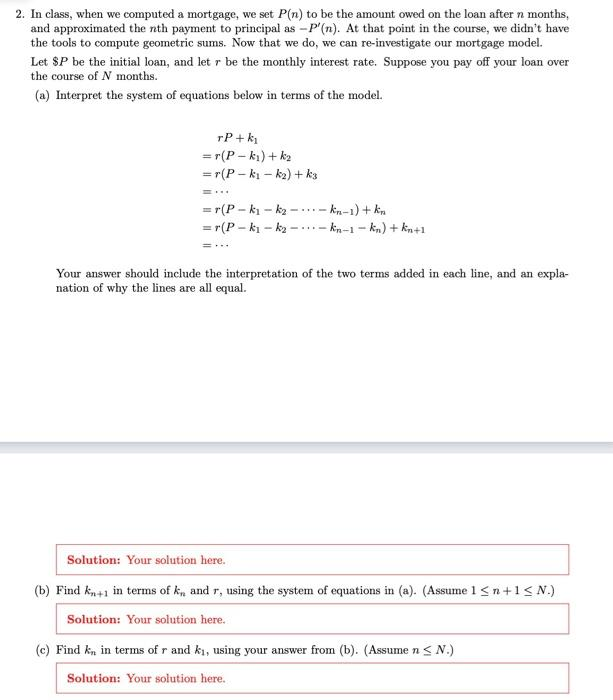# Question 2. In class, when we computed a mortgage, we set $$P(n)$$ to be the amount owed on the loan after $$n$$ months, and approximated the $$n$$th payment to principal as $$-P^{\prime}(n)$$. At that point in the course, we didn't have the tools to compute geometric sums. Now that we do, we can re-investigate our mortgage model. Let $$\ P$$ be the initial loan, and let $$r$$ be the monthly interest rate. Suppose you pay off your loan over the course of $$N$$ months. (a) Interpret the system of equations below in terms of the model. \begin{aligned} & r P+k_{1} \\ = & r\left(P-k_{1}\right)+k_{2} \\ = & r\left(P-k_{1}-k_{2}\right)+k_{3} \\ = & \cdots \\ = & r\left(P-k_{1}-k_{2}-\cdots-k_{n-1}\right)+k_{n} \\ = & r\left(P-k_{1}-k_{2}-\cdots-k_{n-1}-k_{n}\right)+k_{n+1} \\ = & \cdots \end{aligned} Your answer should include the interpretation of the two terms added in each line, and an explanation of why the lines are all equal.Transcribed Image Text: 2. In class, when we computed a mortgage, we set $$P(n)$$ to be the amount owed on the loan after $$n$$ months, and approximated the $$n$$th payment to principal as $$-P^{\prime}(n)$$. At that point in the course, we didn't have the tools to compute geometric sums. Now that we do, we can re-investigate our mortgage model. Let $$\ P$$ be the initial loan, and let $$r$$ be the monthly interest rate. Suppose you pay off your loan over the course of $$N$$ months. (a) Interpret the system of equations below in terms of the model. \begin{aligned} & r P+k_{1} \\ = & r\left(P-k_{1}\right)+k_{2} \\ = & r\left(P-k_{1}-k_{2}\right)+k_{3} \\ = & \cdots \\ = & r\left(P-k_{1}-k_{2}-\cdots-k_{n-1}\right)+k_{n} \\ = & r\left(P-k_{1}-k_{2}-\cdots-k_{n-1}-k_{n}\right)+k_{n+1} \\ = & \cdots \end{aligned} Your answer should include the interpretation of the two terms added in each line, and an explanation of why the lines are all equal.
Transcribed Image Text: 2. In class, when we computed a mortgage, we set $$P(n)$$ to be the amount owed on the loan after $$n$$ months, and approximated the $$n$$th payment to principal as $$-P^{\prime}(n)$$. At that point in the course, we didn't have the tools to compute geometric sums. Now that we do, we can re-investigate our mortgage model. Let $$\ P$$ be the initial loan, and let $$r$$ be the monthly interest rate. Suppose you pay off your loan over the course of $$N$$ months. (a) Interpret the system of equations below in terms of the model. \begin{aligned} & r P+k_{1} \\ = & r\left(P-k_{1}\right)+k_{2} \\ = & r\left(P-k_{1}-k_{2}\right)+k_{3} \\ = & \cdots \\ = & r\left(P-k_{1}-k_{2}-\cdots-k_{n-1}\right)+k_{n} \\ = & r\left(P-k_{1}-k_{2}-\cdots-k_{n-1}-k_{n}\right)+k_{n+1} \\ = & \cdots \end{aligned} Your answer should include the interpretation of the two terms added in each line, and an explanation of why the lines are all equal.&#12304;General guidance&#12305;The answer provided below has been developed in a clear step by step manner.Step1/3(a) The system of equations represents the amortization schedule of a mortgage with an initial loan amount of P and a monthly interest rate of r. Each equation in the system represents the remaining loan balance after making a certain number of payments. The two terms added in each equation represent the interest charged on the remaining balance and the payment made towards the loan principal. The lines are all equal because they represent the same remaining loan balance, just expressed in terms of different numbers of payments.Explanation:The lines are all equal because they represent the same remaining loan balance, just expressed in terms of different numbers of payments.Explanation:Please refer to solution in this step.Step2/3(b) We can use the system of equations to solve for $$\mathrm{{k}_{{{n}+{1}}}}$$. Starting from the first equation, we have:$$\mathrm{{r}{P}+{k}_{{{1}}}={r}{\left({P}-{k}_{{{1}}}\right)}+{k}_{{{2}}}}$$Simplifying this equation gives:$$\mathrm{{k}_{{{1}}}={r}{\left({P}-{2}{k}_{{{1}}}\right)}+{k}_{{{2}}}}$$Substituting this into the second equation gives:$$\mathrm{{r}{\left({P}-{k}_{{{1}}}-{k}_{{{2}}}\right)}+{k}_{{{3}}}={r}{\left({P}-{k}_{{{1}}}-{2}{k}_{{{2}}}\right)}+{k}_{{{3}}}+{k}_{{{2}}}}$$Simplifying this equation gives:$$\mathrm{{k}_{{{2}}}={\left({1}+{r}\right)}{\left({P}-{k}_{{{1}}}\right)}-{k}_{{{3}}}}$$Substituting this into the third equation gives:$$\mathrm{{r}{\left({P}-{k}_{{{1}}}-{k}_{{{2}}}-{k}_{{{3}}}\right)}+{k}_{{{4}}}={r}{\left({P}-{k}_{{{1}}}-{2}{k}_{{{2}}}-{k}_{{{3}}}\right)}+{k}_{{{4}}}+{k}_{{{3}}}}$$Simplifying this equation gives:$$\mathrm{{k}_{{{3}}}={\left({1}+{r}\right)}{\left({P}-{k}_{{{1}}}-{k}_{{{2}}}\right)}-{k}_{{{4}}}}$$Continuing in this way, we can derive the following expressi ... See the full answer